Test: Basic Geometrical Ideas

# Test: Basic Geometrical Ideas

Test Description

## 10 Questions MCQ Test NCERT Mathematics for CAT Preparation | Test: Basic Geometrical Ideas

Test: Basic Geometrical Ideas for Class 6 2022 is part of NCERT Mathematics for CAT Preparation preparation. The Test: Basic Geometrical Ideas questions and answers have been prepared according to the Class 6 exam syllabus.The Test: Basic Geometrical Ideas MCQs are made for Class 6 2022 Exam. Find important definitions, questions, notes, meanings, examples, exercises, MCQs and online tests for Test: Basic Geometrical Ideas below.
Solutions of Test: Basic Geometrical Ideas questions in English are available as part of our NCERT Mathematics for CAT Preparation for Class 6 & Test: Basic Geometrical Ideas solutions in Hindi for NCERT Mathematics for CAT Preparation course. Download more important topics, notes, lectures and mock test series for Class 6 Exam by signing up for free. Attempt Test: Basic Geometrical Ideas | 10 questions in 10 minutes | Mock test for Class 6 preparation | Free important questions MCQ to study NCERT Mathematics for CAT Preparation for Class 6 Exam | Download free PDF with solutions
 1 Crore+ students have signed up on EduRev. Have you?
Test: Basic Geometrical Ideas - Question 1

### How many points does the given figure has?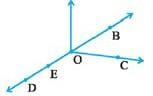Detailed Solution for Test: Basic Geometrical Ideas - Question 1

(B,C,D,E,O)5 POINTS

Test: Basic Geometrical Ideas - Question 2

### In the given figure, the ray will be named as _____.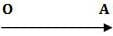Detailed Solution for Test: Basic Geometrical Ideas - Question 2

We start from the letter which has an exact point (O here) and then we write the letter which is extended infinitely (A here) hence OA

Test: Basic Geometrical Ideas - Question 3

### Which of the following is an open curve?

Detailed Solution for Test: Basic Geometrical Ideas - Question 3

Rest of the figures are totally closed but only figure D is open .

Test: Basic Geometrical Ideas - Question 4

The line segment forming a polygon are called _________________.

Detailed Solution for Test: Basic Geometrical Ideas - Question 4

The line segments that form a polygon are called the sides of the polygon. A point where two sides meet is a vertex (plural form is vertices).

Test: Basic Geometrical Ideas - Question 5

Two distinct lines meeting at a points are called _____________.

Detailed Solution for Test: Basic Geometrical Ideas - Question 5

Intersecting Lines: Two lines which meet at one point are said to be intersecting lines. The common point is called the ‘point of intersection’.

Test: Basic Geometrical Ideas - Question 6

How many lines pass through one given point?

Detailed Solution for Test: Basic Geometrical Ideas - Question 6

Countless or Infinite number of lines can pass through a single point.

Test: Basic Geometrical Ideas - Question 7

Name the point intersection in the given figure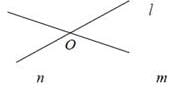Detailed Solution for Test: Basic Geometrical Ideas - Question 7

These two lines meet at point O and hence it is called the intersection point of the two lines.

Test: Basic Geometrical Ideas - Question 8

How many lines pass through two given points?

Detailed Solution for Test: Basic Geometrical Ideas - Question 8

Only one line can pass through two given points.

Test: Basic Geometrical Ideas - Question 9

Which of the following is pair of opposite sides in the given figure?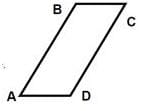Test: Basic Geometrical Ideas - Question 10

Which of the following is the pair of adjacent angles in the given figure ?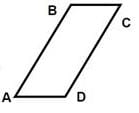Detailed Solution for Test: Basic Geometrical Ideas - Question 10

The angles which have a ray in common are called adjacent angles. Here A and B have AB as common and hence they are adjacent.

## NCERT Mathematics for CAT Preparation

276 docs|149 tests
 Use Code STAYHOME200 and get INR 200 additional OFF Use Coupon Code
Information about Test: Basic Geometrical Ideas Page
In this test you can find the Exam questions for Test: Basic Geometrical Ideas solved & explained in the simplest way possible. Besides giving Questions and answers for Test: Basic Geometrical Ideas, EduRev gives you an ample number of Online tests for practice

## NCERT Mathematics for CAT Preparation

276 docs|149 tests